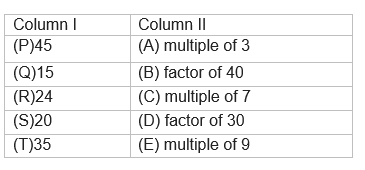# Class 6 Maths Playing With Numbers MCQ

In this page we have Multiple Choice questions for Class 6 Maths Playing With Numbers . Hope you like them and do not forget to like , social share and comment at the end of the page.

## Multiple Choice Questions

Question 1
Which of these is the factor of 50.
a. 10
b. 3
c. 7
d. 6
Question 2
What is the Sixth multiple of 13?
a. 78
b. 65
c. 52
d. 91
Question 3
Which of them is a prime number?
a. 13
b. 14
c. 28
d. 25
Question 4
Which of them is a composite number?
a. 45
b. 11
c. 31
d. 13
Question 5
The number of multiples of a given number is
a. 10
b. 100
c. 1000
d. infinite
Question 6
The smallest composite number is
a. 4
b. 1
c. 9
d. 6
Question 7
What are Two numbers called having only 1 as a common factor.
a. co-prime numbers
b. twin prime numbers
c. composite numbers
d. prime numbers.
Question 8
The HCF of two co-prime numbers is
a. 0
b. 3
c. 2
d. 1
Question 9
The HCF of 12 and 16 is
a. 2
b. 4
c. 6
d. 1
Question 10
The LCM of 12 and 16 is
a. 24
b. 48
c. 96
d. 32

Question 11
The HCF of two consecutive numbers is
a. 0
b. 1
c. 2
d. 3
Question 12
The HCF of two consecutive odd numbers is
a. 3
b. 2
c. 1
d. 0
Question 13
The HCF of two consecutive even numbers is
a. 0
b. 1
c. 2
d. 3
Question 14
The HCF of 66,77,88
a. 11
b. 12
c. 13
d. 17
Question 15
The LCM of two consecutive numbers is
a. their sum
b. their product
c. their difference
d. none of these
Question 16
The smallest odd composite number is
a. 5
b. 15
c. 9
d. 3
Question 17
Find the smallest 4-digit number which is divisible by 6, 8, 9
a. 2012
b. 2160
c. 2088
d. 2016
Question 18
The LCM of two co-prime numbers is
a. their sum
b. their product
c. their difference
d. none of these
Question 19
Which of the following is false?
a. The number 2 and 3 are co-prime
b. The numbers 41 and 43 are twin primes
c. The number 5,28   are co-prime
d. none of these
Question 20
Match the items in column I and column II.Which of the following is true?
(a)  P -> E, Q -> D,R-> A, S-> B,T->C
(b)  P -> D, Q -> E,R-> A, S-> A,T->C
(c)  P -> E, Q -> D,R-> B, S-> A,T->C
(d)  P -> E, Q -> D,R-> A, S-> C,T->B

(1)a
(2) a
(3) a
(4) a
(5) d
(6) a
(7) a
(8) d
(9) b
(10) b
(11) b
(12) c
(13) c
(14) a
(15) b
(17) d
(18) b
(19) d
(20) a### Practice Question

Question 1 What is $\frac {1}{2} + \frac {3}{4}$ ?
A)$\frac {5}{4}$
B)$\frac {1}{4}$
C)$1$
D)$\frac {4}{5}$
Question 2 Pinhole camera produces an ?
A)An erect and small image
B)an Inverted and small image
C)An inverted and enlarged image
D)None of the above